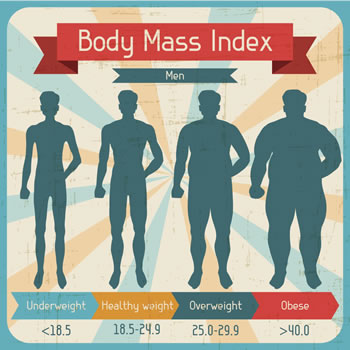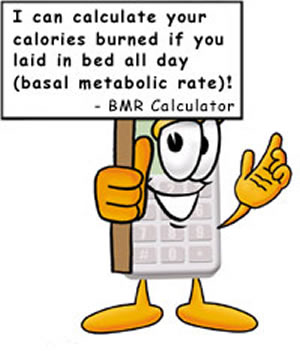# Difference between BMI and BMR

Key Difference: BMI is the statistical measurement of a person’s current body weight in relevance to height. BMR is the number of calories a person should be consuming every day even if they haven’t done much apart from lying in bed doing nothing.BMI and BMR are two methods that are commonly used by someone trying to maintain fitness or lose weight. However, the two methods are completely different and used for two different reasons.

BMI stands for body mass index. It is a body measurement technique that allows one to know whether they are underweight, ideal weight or overweight. BMI is an index, which means that it shows a ratio. In this case, BMI is the ratio between one’s height and weight. BMI is calculated as mass(kg)/height(m)2. It can also be calculated as (mass(lb)/height(in)2)x703. Alternately, BMI is also often calculated on the basis of a table or chart, which usually has different BMI categories segregated on it. There are many online BMI calculators available that automate the calculation process.

Depending on the answer, one falls into either underweight, ideal weight or overweight categories. The different categories are:

• BMI of less than 15 is considered very severely underweight
• BMI in-between 15.0 – 15.9 is considered severely underweight
• BMI in-between 16.0 – 18.4 is considered underweight
• BMI in-between 18.5 – 24.9 is considered normal weight
• BMI in-between 25.0 – 29.9 is considered overweight
• BMI in-between 30.0 – 34.9 is considered class I obesity
• BMI in-between 35.0 – 39.9 is considered class II obesity
• BMI of more than 40.0 is considered class III obesity

BMR, which stands for basal metabolic rate, is a biological process. It indicates the amount of energy that is expended by someone while at rest. This energy is what is normally required to run the basic functioning of the vital organs, such as the heart, lungs, nervous system, kidneys, liver, intestine, sex organs, muscles, brain, and skin. As the BMR is energy, it is often reported in units of kJ, in this case kJ per hour per kg body mass.

BMR is the least required energy by the body. If the body just more, such as exercise then the body would require more energy, to compensate for the additional energy expended. BMR is a key technique used for weight loss. However, BMR is harder to calculate, and the calculations differ slightly for males and females. The easiest way to calculate BMR is to use an online calculator.The rule of thumb is the 3500 calories equals to one pound. This means that in order to lose 1 lb (0.45 kg), one must create a deficit of 3500 calories. The BMR tells one how much they need to eat to allow the body to function, if they eat more than that, then the body converts the additional calories into fat. However, if one eats less than that, then the body will tap into the fat reserves for the additional calories needed.

The main difference between BMI and BMR is that BMI is the statistical measurement of a person’s current body weight in relevance to height. BMR, on the other hand, is the number of calories a person should be consuming every day even if they haven’t done much apart from lying in bed doing nothing. BMI has been criticized by many, as it does not take anything, such as body composition, or age, into account. It is solely based on height and weight. Whereas BMR is individualized person to person; it also takes weight, height and age into account.

Comparison between BMI and BMR:

 BMI BMR Full Form Body Mass Index Basal Metabolic Rate Description The individual's body mass divided by the square of their height. The rate of energy expenditure at rest Methods Via formula, a table or chart, or an online calculator. Via formula, direct or indirect calorimetry, or through an online calculator. Gender Uses the same method and formula for any gender. Uses different formulas for male and female. Age Constant at any age BMR decreases with age Formula mass(kg) height(m)2   or   (mass(lb)    x703 height(in)2) Men: 88.362 + ( 13.397 x weight in kg ) + ( 4.799 x height in cm ) - ( 5.677 x age in years)   Women: 447.593 + ( 9.247 x weight in kg ) + ( 3.098 x height in cm ) - (4.330 x age in years)
###### مرورگر شما بسیار قدیمی است!
جهت مشاهده این وب سایت به صورت صحیح، بروزرسانی مرورگرتان ضروری خواهد بود. بروزرسانی مرورگر
×